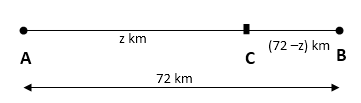# Time and Distance - Aptitude Questions and Answers

## Time and Distance Questions and Answers

Learn and practice the chapter "Time and Distance" with these solved Aptitude Questions and Answers. Each question in the topic is accompanied by a clear and easy explanation, diagrams, formulae, shortcuts and tricks that help in understanding the concept.

## Use of Time and Distance Questions

The questions and examples given in this section will be useful to all the freshers, college students and engineering students preparing for placement tests or any competitive exam like MBA, CAT, MAT, SNAP, MHCET, XAT, NMAT, GATE, Bank exams - IBPS, SBI, RBI, RRB, SSB, SSC, UPSC etc.

Practice with this online test to crack your placements and entrance tests!
1. To reach school half an hour early, Meera has to increase her speed to 7/4 of her usual speed. How much time does she take every day to reach the school?

a. 54 min
b. 66.67 min
c. 67.50 min
d. 70 min

Explanation:

D = S x T
 ∴ S = D ; T = D T S

We know, Distance travelled is same both times.
∴ D = D
 ∴ S x T = 7S x (T-30) 4
∴ 4T = 7T - 210
∴ T = 70 min

## Video : Speed, Distance and Time in Hindi - Simple Aptitude tricks for freshers

2. Two friends Amar and Brijesh start from two different points and walk towards each other in a straight line. After meeting each other somewhere on the way, they finish their travel in 324 hours and 225 hours respectively. What is the ratio of speed of Amar to that of Brijesh?

a. 15:18
d. 18:15
c. 225:324
d. 324:225

Explanation:

Tip:
If two bodies start moving towards each other at the same time from points A and B and on crossing each other, if they take X and Y hours in reaching B and A respectively, then -
Speed of A : Speed of B =  Y : X

Using the above standard formula -
X = 324 hours
Y = 225 hours
 Speed of A : Speed of B = Y : X = Y = 225 = 15 X 324 18

3. Two cities Alipur and Balipur are 72 km apart. Arun and Varun who stay at Alipur start riding on bicycle to Balipur. Arun travels at a speed of 17 kmph while Varun’s speed is 2 kmph more than Arun. Varun was riding faster, so he reaches Balipur early and returns immediately. On his way back he meets Arun at Chandipur . How far is Chandipur from Balipur?

a. 4 km
b. 10 km
c. 15 km
d. 36 km

Explanation:

Tip:
Understanding and solving such problems is very easy by drawing a line.To make things easy, let's set some notations:
Alipur - A ; Balipur - B; Chandipur - C

Let distance between A and C be 'z'
∴ Distance between B and C = 72 – z
Distance travelled by Arun = AC = z km
Distance travelled by Varun = AB + BC = (72+(72-z)) = (144-z) km

D = S x T
 ∴ S = D ; T = D T S

We know, time for both is same
∴ T = T
 ∴ z = 144-z 17 19
∴ z = 68 km
∴ Distance between B and C = 72 - z = 72 - 68 = 4 km

Now, if it is asked to calculate total distance travelled by Varun before meeting Arun, do as follows -
Distance travelled by Varun before meeting Arun = AB + BC = 72 + 4 = 76 km

4. A dog sees a cat 80 m away. The cat runs at a speed of 5 m/s while the dog chases it at a speed 2 m/s more than that of cat. Before the dog is able to catch the cat, how much distance has it already run?

a. 50 m
b. 100 m
c. 130 m
d. 200 m

Explanation:

D = S x T
 ∴ S = D ; T = D T S

Let distance travelled by cat before dog catches it be D
We know, time for which Dog and Cat ran is same
∴ T = T
 ∴ D = D+80 5 7
∴ D = 200 m

5. Ramesh says, "Driving at an average speed of 60 kmph, I reach office 10 minutes early. However, if I drive at a speed 10 kmph lesser than the earlier, I get late by half an hour". Find the distance between Ramesh's office and home.

a. 60 km
b. 80 km
c. 90 km
d. 100 km

Explanation:

D = S x T
 ∴ S = D ; T = D T S

Let distance be D
With speed 50km/hr (10 kmph less than the earlier 60 kmph), he is 30 minutes late
With speed 60 km/hr he is 10 minutes early
 ∴ Difference between two times = 30+10 = 40min = 40 hours 60

Tip:
Difference between two given times can also be easily measured or checked by looking at a watch or imagining a watch.

 Also, time = T = D S
 ∴ D - D = 40 50 60 60
∴ D = 80 km